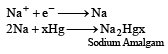Courses

# Test: The S - Block Elements - From Past 28 Years Questions

## 38 Questions MCQ Test Chemistry Class 11 | Test: The S - Block Elements - From Past 28 Years Questions

Description
This mock test of Test: The S - Block Elements - From Past 28 Years Questions for NEET helps you for every NEET entrance exam. This contains 38 Multiple Choice Questions for NEET Test: The S - Block Elements - From Past 28 Years Questions (mcq) to study with solutions a complete question bank. The solved questions answers in this Test: The S - Block Elements - From Past 28 Years Questions quiz give you a good mix of easy questions and tough questions. NEET students definitely take this Test: The S - Block Elements - From Past 28 Years Questions exercise for a better result in the exam. You can find other Test: The S - Block Elements - From Past 28 Years Questions extra questions, long questions & short questions for NEET on EduRev as well by searching above.
QUESTION: 1

### Which one of the following properties of alkali metals increases in magnitude as the atomic number rises ? 

Solution:

Within a group, ionic radius increases with increase in atomic number. The melting points decrease down the group due to weakning of metallic bond. The electronegativity and the 1st ionization energy also decreases down the group.

QUESTION: 2

### Wh ich of the following atoms will have the smallest size ? 

Solution:

Within a period, the atomic size decreases from left to right.Further atomic size increases down the group. Hence the correct order is   i,e. Na > Mg > Li > Be.

QUESTION: 3

### Compared with the alkaline earth metals, the alkali metals exhibit 

Solution:

Because of larger size and smaller nuclear charge, alkali metals have low ionization potential relative to alkaline earth metals.

QUESTION: 4

Washing soda has formula 

Solution:

Washing soda is Na2 CO3. 10 H2O.

QUESTION: 5

Electronic configuration of calcium atom may be written as 

Solution:

Ca(20) = 1s22s23p6 3s23p6 4s2 = [Ar] 4s2

QUESTION: 6

Which of the following will react most vigorously with water

Solution:

Out of Li, Na, K and Rb, Rb reacts fastest with water. The rate of reaction increases down the group.

QUESTION: 7

Which one of the following has minimum value of cation/anion ratio. 

Solution:

Atomic size of K+ > Ca2+ > Mg2+ and that of Cl > F. Therefore, Mg2+/Cl ratio has the minimum value.

QUESTION: 8

Which of the following has largest size ? 

Solution:

A cation is always much smaller than the corresponding atom(with increase in positive charge, atomic particles move close to each other), whereas an anion is always larger than the corresponding atom(with increase in negative charge, size increases to minimise the effect of steric repulsion).

QUESTION: 9

Which of the following is known as fusion mixture? 

Solution:

Mixture of K2CO3 and Na2CO3 is called fusion mixture

QUESTION: 10

All the following substances react with water.The pair that gives the same gaseous product is 

Solution:

Ca and CaH2 both react with H2O to form H2 gas,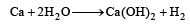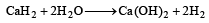whereas K gives H2 while KO2 gives O2 and H2O2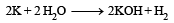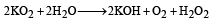Similarly, Na gives H2 while Na2 O2 gives
H2O2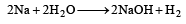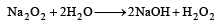Likewise Ba gives H2 while BaO2 gives H2O2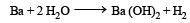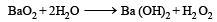QUESTION: 11

Among the following oxides, the one which is most basic is 

Solution:

MgO. N2O5 is strongly acidic, ZnO and Al2 O3 are amphoteric, therefore, MgO is most basic.

QUESTION: 12

Which of the following metal ions plays an important role in muscle contraction ? 

Solution:

Ca2+ ions is an essential element for the contraction of muscles.

QUESTION: 13

Which of the following statement is false ? 

Solution:

Be(OH)2 is amphoteric, but the hydroxides of other alkaline earth metals are basic. The basic strength increases gradually.

QUESTION: 14

Sodium is made by the electrolysis of a molten mixture of about 40% NaCl and 60% CaCl2 because 

Solution:

Sodium is obtained by electrolytic reduction of its chloride. Melting point of chloride of sodium is high (803°C) so in order to lower its melting point(600°C), calcium chloride is added to it.

QUESTION: 15

Identify the correct statement 

Solution:

Gypsum is CaSO4. 2H2O and plaster of Paris i s (CaSO4)2 .H2O. Therefore gypsum contains a lower percentage of calcium than plaster of Paris.

QUESTION: 16

Aqueous solution of sodium carbonate absorbs NO and NO2 to give 

Solution: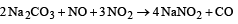QUESTION: 17

Which one is the correct statement with reference to solubility of MgSO4 in water? 

Solution:

MgSO4 is the only alkaline ear th metal sulphate which is soluble in water and for solubility hydration energy should be greater than lattice energy i.e hydration energy > lattice energy

QUESTION: 18

Calcium is obtained by the 

Solution:

Calcium is obtained by electrolysis of a fused mass consisting six parts calcium chloride and one part calcium fluoride at about 700°C in an electrolytic cell.

QUESTION: 19

In crystals of which one of the following ionic compounds would you expect maximum distance between centres of cations and anions? 

Solution:

As Cs+ ion has larger size than Li+ and I has larger size than F, therefore maximum distance between centres of cations and anions is in CsI.

QUESTION: 20

In which of the following processes, fused sodium hydroxide is electrolysed at a 330ºC temperature for extraction of sodium? 

Solution:

In Castner process, for production of (Na) Sodium metal, Sodium hydroxide (NaOH) is electrolysed at temperature 330ºC.

QUESTION: 21

A solid compound ‘X’ on heating gives CO2 gas and a residue. The residue mixed with water forms ‘Y’. On passing an excess of CO2 through ‘Y’ in water, a clear solution ‘Z’, is obtained. On boiling ‘Z’, a compound ‘X’ is reformed. The compound ‘X’ is

Solution: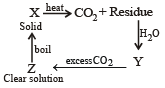The given properties coincide with CaCO3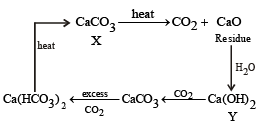QUESTION: 22

The correct order of the mobility of the alkali metal ions in aqueous solutions is 

Solution:

Hydration enthalpies of alkali metal ions decreases with the increase in ionic radii as we move down the group.

Therefore, smaller is the mass of hydrated species higher is the ionic mobility in the aqueous solution.

Thus, the order for ionic mobility of ions is

Rb+ > K+ > Na+ > Li+

QUESTION: 23

The correct order of increasing thermal stability of K2CO3, MgCO3, CaCO3 and BeCO3 is

Solution:

As the basicity of metal hydroxides increases down the group from Be to Ba, the thermal stability of their carbonates also increases in the same order. Further group 1 compounds are more thermally stable than group 2 because their hydroxide are much basic than group 2 hydroxides therefore, the order of thermal stability is BeCO3 < MgCO3< CaCO3< K2CO3.

QUESTION: 24

In which of the following the hydration energy is higher than the lattice energy? 

Solution:

The solubility of sulphates of alkaline earth metals decreases as we move down the group from Be to Ba due to the reason that ionic size increases down the group. The lattice energy remains constant because sulphate  ion is so large, so that small change in cationic sizes do not make any difference.
Thus the order: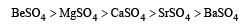QUESTION: 25

The sequence of ioinic mobility in aqueous solution is : 

Solution:

Smaller the ion more is its ionic mobility in aqueous solution. Ionic radii of the given alkali metals is in the order Na+ < K+ < Rb+ < Cs+ and thus expected ionic mobility will be in the order Cs+ < Rb+ < K+ < Na+. However due to high degree of solvation (or hydration) because of lower size or high charge density, the hydrated ion size follows the order Cs+ < Rb+ < K+ < Na+ and thus conductivity order is Cs> Rb+ > K+ > Na+ i.e. option (b) is correct answer.

QUESTION: 26

The alkali metals form salt-like hydrides by the direct synthesis at elevated temperature. The thermal stability of these hydrides decreases in which of the following orders ? 

Solution:

The stability of alkali metal hydrides decreases from Li to Cs. It is due to the  fact that M–H bonds becomes weaker with increase in size of alkali metals as we move down the group from Li to Cs. Thus the order of stability of hydrides is LiH > NaH > KH > RbH > CsH i.e. option (d) is correct answer.

QUESTION: 27

Which of the following oxides is not expected to react with sodium hydroxide? 

Solution:

NaOH is a strong alkali. It combines with acidic and amphoteric oxides to form salts.
Since CaO is a basic oxide hence does not reacts with NaOH.

QUESTION: 28

Which of the following alkaline earth metal sulphates has hydration enthalpy higher than the lattice enthalpy? 

Solution:

Be2+ is very small, hence its hydration enthalpy is greater than its lattice enthalpy (At. Nos. Mn = 25, Fe = 26, Co = 27, Ni = 28)

QUESTION: 29

Property of the alkaline earth metals that increases with their atomic number : 

Solution:

Lattice energy decreases more rapidly than hydration energy for alkaline earth metal hydroxides.

QUESTION: 30

The compound A on heating gives a colourless gas and a residue that is dissolved in water to obtain B. Excess of CO2 is bubbled through aqueous solution of B, C is formed which is recovered in the solid form. Solid C on gentle heating gives back A. The compound is 

Solution: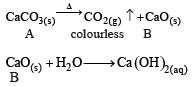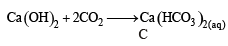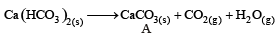∴ Correct choice : (b)

QUESTION: 31

Which of the following compounds has the lowest melting point ? 

Solution:

Melting points of halides decreases as the size of the halogen increases. The correct order is CaF2 > CaCl2 > CaBr2 > CaI2

QUESTION: 32

Which one of the following is present as an active ingredient in bleaching powder for bleaching action ? 

Solution:

Active ingredient in bleaching powder for bleaching action is Ca (OCl)2

QUESTION: 33

Which of the following statements is incorrect ? [2011M]

Solution: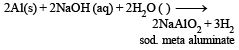QUESTION: 34

Match List – I with List –II for the compositions of substances and select the correct answer using the code given below the lists : [2011M]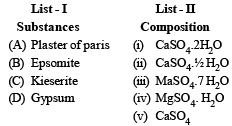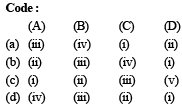Solution:

(A) Plaster of paris =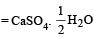(B) Epsomite = MgSO4.7H2O
(C) Kieserite = MgSO4.H2O
(D) Gypsum = CaSO4.2H2O

QUESTION: 35

Which one of the alkali metals, forms only, the normal oxide, M2O on heating in air ? 

Solution:

All the alkali metals when heated with oxygen form different types of oxides for example lithium forms lithium oxide (Li2O), sodium forms sodium peroxide (Na2O2), while K, Rb and Cs form their respective superoxides.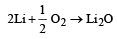QUESTION: 36

The ease of adsorption of the hydrated alkali metal ions on an ion-exchange resins follows the order :

Solution:

All a lkali  metal salts are ionic (except Lithium) and soluble  in water due to the fact that cations get hydrated by water molecules. The  degree of hydration depends upon the size of the cation. Smaller the size of a cation, greater is its hydration energy.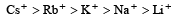Relative ionic radii in water or relative degree of hydration: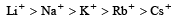QUESTION: 37

In the replacement reaction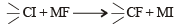The reaction will be most favourable if M happens to be : [2012 M]

Solution:

Tertiary halide can show ionic reaction with MF so, MF should be most ionic for reaction to proceed forward. Hence ‘M’ should be ‘Rb’.

QUESTION: 38

In Castner-Kellner cell for production of sodium hydroxide: [NEET Kar. 2013]

Solution:

At the cathode, since the discharge + ions is lower than that of H+ ions on the mercury, cathode, so Na+ ions are discharged while H+ ions remain in the solution.